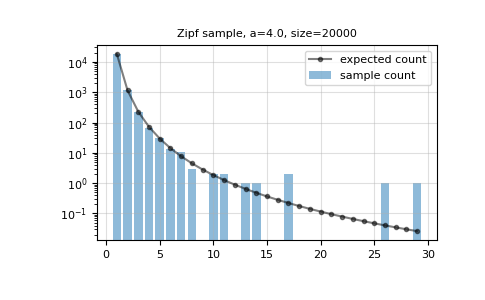#### Previous topic

numpy.random.weibull

Bit Generators

# numpy.random.zipf¶

numpy.random.zipf(a, size=None)

Draw samples from a Zipf distribution.

Samples are drawn from a Zipf distribution with specified parameter a > 1.

The Zipf distribution (also known as the zeta distribution) is a continuous probability distribution that satisfies Zipf’s law: the frequency of an item is inversely proportional to its rank in a frequency table.

Note

New code should use the zipf method of a default_rng() instance instead; see random-quick-start.

Parameters
afloat or array_like of floats

Distribution parameter. Must be greater than 1.

sizeint or tuple of ints, optional

Output shape. If the given shape is, e.g., (m, n, k), then m * n * k samples are drawn. If size is None (default), a single value is returned if a is a scalar. Otherwise, np.array(a).size samples are drawn.

Returns
outndarray or scalar

Drawn samples from the parameterized Zipf distribution.

scipy.stats.zipf

probability density function, distribution, or cumulative density function, etc.

Generator.zipf

which should be used for new code.

Notes

The probability density for the Zipf distribution iswhereis the Riemann Zeta function.

It is named for the American linguist George Kingsley Zipf, who noted that the frequency of any word in a sample of a language is inversely proportional to its rank in the frequency table.

References

1

Zipf, G. K., “Selected Studies of the Principle of Relative Frequency in Language,” Cambridge, MA: Harvard Univ. Press, 1932.

Examples

Draw samples from the distribution:

>>> a = 2. # parameter
>>> s = np.random.zipf(a, 1000)


Display the histogram of the samples, along with the probability density function:

>>> import matplotlib.pyplot as plt
>>> from scipy import special


Truncate s values at 50 so plot is interesting:

>>> count, bins, ignored = plt.hist(s[s<50], 50, density=True)
>>> x = np.arange(1., 50.)
>>> y = x**(-a) / special.zetac(a)
>>> plt.plot(x, y/max(y), linewidth=2, color='r')
>>> plt.show()